The Normal Distribution

# 32 Estimating the Binomial with the Normal Distribution

We found earlier that various probability density functions are the limiting distributions of others; thus, we can estimate one with another under certain circumstances. We will find here that the normal distribution can be used to estimate a binomial process. The Poisson was used to estimate the binomial previously, and the binomial was used to estimate the hypergeometric distribution.

In the case of the relationship between the hypergeometric distribution and the binomial, we had to recognize that a binomial process assumes that the probability of a success remains constant from trial to trial: a head on the last flip cannot have an effect on the probability of a head on the next flip. In the hypergeometric distribution this is the essence of the question because the experiment assumes that any “draw” is without replacement. If one draws without replacement, then all subsequent “draws” are conditional probabilities. We found that if the hypergeometric experiment draws only a small percentage of the total objects, then we can ignore the impact on the probability from draw to draw.

Imagine that there are 312 cards in a deck comprised of 6 normal decks. If the experiment called for drawing only 10 cards, less than 5% of the total, than we will accept the binomial estimate of the probability, even though this is actually a hypergeometric distribution because the cards are presumably drawn without replacement.

The Poisson likewise was considered an appropriate estimate of the binomial under certain circumstances. In Chapter 4 we found that if the number of trials of interest is large and the probability of success is small, such that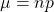<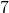, the Poisson can be used to estimate the binomial with good results. Again, these rules of thumb do not in any way claim that the actual probability is what the estimate determines, only that the difference is in the third or fourth decimal and is thus de minimus.

Here, again, we find that the normal distribution makes particularly accurate estimates of a binomial process under certain circumstances. (Figure) is a frequency distribution of a binomial process for the experiment of flipping three coins where the random variable is the number of heads. The sample space is listed below the distribution. The experiment assumed that the probability of a success is 0.5; the probability of a failure, a tail, is thus also 0.5. In observing (Figure) we are struck by the fact that the distribution is symmetrical. The root of this result is that the probabilities of success and failure are the same, 0.5. If the probability of success were smaller than 0.5, the distribution becomes skewed right. Indeed, as the probability of success diminishes, the degree of skewness increases. If the probability of success increases from 0.5, then the skewness increases in the lower tail, resulting in a left-skewed distribution.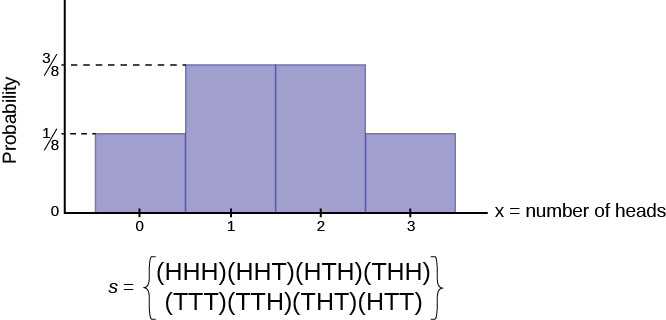The reason the skewness of the binomial distribution is important is because if it is to be estimated with a normal distribution, then we need to recognize that the normal distribution is symmetrical. The closer the underlying binomial distribution is to being symmetrical, the better the estimate that is produced by the normal distribution. (Figure) shows a symmetrical normal distribution transposed on a graph of a binomial distribution where p = 0.2 and n = 5. The discrepancy between the estimated probability using a normal distribution and the probability of the original binomial distribution is apparent. The criteria for using a normal distribution to estimate a binomial thus addresses this problem by requiring BOTH np AND n(1 − p) are greater than five. Again, this is a rule of thumb, but is effective and results in acceptable estimates of the binomial probability.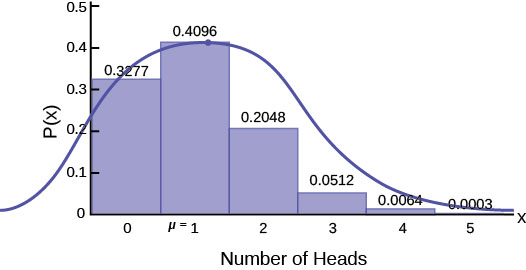Imagine that it is known that only 10% of Australian Shepherd puppies are born with what is called “perfect symmetry” in their three colors, black, white, and copper. Perfect symmetry is defined as equal coverage on all parts of the dog when looked at in the face and measuring left and right down the centerline. A kennel would have a good reputation for breeding Australian Shepherds if they had a high percentage of dogs that met this criterion. During the past 5 years and out of the 100 dogs born to Dundee Kennels, 16 were born with this coloring characteristic.

What is the probability that, in 100 births, more than 16 would have this characteristic?

If we assume that one dog’s coloring is independent of other dogs’ coloring, a bit of a brave assumption, this becomes a classic binomial probability problem.

The statement of the probability requested is 1 − [p(X = 0) + p(X = 1) + p(X = 2)+ … + p(X = 16)]. This requires us to calculate 17 binomial formulas and add them together and then subtract from one to get the right hand part of the distribution. Alternatively, we can use the normal distribution to get an acceptable answer and in much less time.

First, we need to check if the binomial distribution is symmetrical enough to use the normal distribution. We know that the binomial for this problem is skewed because the probability of success, 0.1, is not the same as the probability of failure, 0.9. Nevertheless, both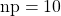and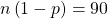are larger than 5, the cutoff for using the normal distribution to estimate the binomial.

(Figure) below shows the binomial distribution and marks the area we wish to know. The mean of the binomial, 10, is also marked, and the standard deviation is written on the side of the graph: σ =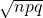= 3. The area under the distribution from zero to 16 is the probability requested, and has been shaded in. Below the binomial distribution is a normal distribution to be used to estimate this probability. That probability has also been shaded.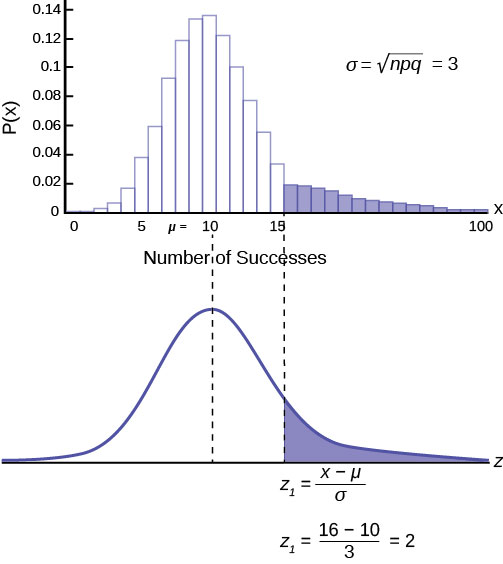Standardizing from the binomial to the normal distribution as done in the past shows where we are asking for the probability from 16 to positive infinity, or 100 in this case. We need to calculate the number of standard deviations 16 is away from the mean: 10.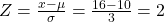We are asking for the probability beyond two standard deviations, a very unlikely event. We look up two standard deviations in the standard normal table and find the area from zero to two standard deviations is 0.4772. We are interested in the tail, however, so we subtract 0.4772 from 0.5 and thus find the area in the tail. Our conclusion is the probability of a kennel having 16 dogs with “perfect symmetry” is 0.0228. Dundee Kennels has an extraordinary record in this regard.

Mathematically, we write this as: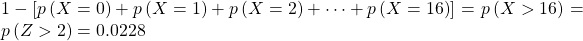### Chapter Review

The normal distribution, which is continuous, is the most important of all the probability distributions. Its graph is bell-shaped. This bell-shaped curve is used in almost all disciplines. Since it is a continuous distribution, the total area under the curve is one. The parameters of the normal are the mean µ and the standard deviation σ. A special normal distribution, called the standard normal distribution is the distribution of z-scores. Its mean is zero, and its standard deviation is one.

### Formula Review

Normal Distribution: X ~ N(µ, σ) where µ is the mean and σ is the standard deviation.

Standard Normal Distribution: Z ~ N(0, 1).

How would you represent the area to the left of one in a probability statement?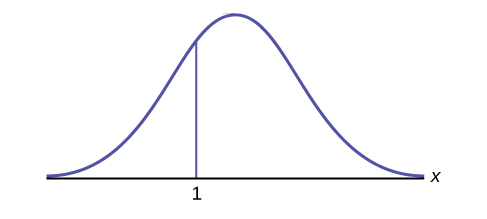P(x < 1)

What is the area to the right of one?<!– <solution id=”fs-idp151140256″> 1 – P(x < 1) or P(x < 1) –>

Is P(x < 1) equal to P(x ≤ 1)? Why?

Yes, because they are the same in a continuous distribution: P(x = 1) = 0

How would you represent the area to the left of three in a probability statement?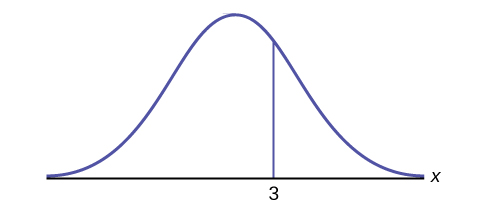<!– <solution id=”fs-idp146985792″> P(x < 3) –>

What is the area to the right of three?1 – P(x < 3) or P(x > 3)

If the area to the left of x in a normal distribution is 0.123, what is the area to the right of x?

<!– <solution id=”fs-idm19166096″> 1 – 0.123 = 0.877 –>

If the area to the right of x in a normal distribution is 0.543, what is the area to the left of x?

1 – 0.543 = 0.457

Use the following information to answer the next four exercises:

X ~ N(54, 8)

Find the probability that x > 56.

<!– <solution id=”fs-idp74510448″> 0.4013 –>

Find the probability that x < 30.

0.0013

X ~ N(6, 2)

Find the probability that x is between three and nine.

<!– <solution id=”fs-idp53207040″> 0.8664 –>

X ~ N(–3, 4)

Find the probability that x is between one and four.

0.1186

X ~ N(4, 5)

Find the maximum of x in the bottom quartile.

<!– <solution id=”fs-idp30677232″> 0.6276 –>

Use the following information to answer the next three exercise: The life of Sunshine CD players is normally distributed with a mean of 4.1 years and a standard deviation of 1.3 years. A CD player is guaranteed for three years. We are interested in the length of time a CD player lasts. Find the probability that a CD player will break down during the guarantee period.

1. Sketch the situation. Label and scale the axes. Shade the region corresponding to the probability.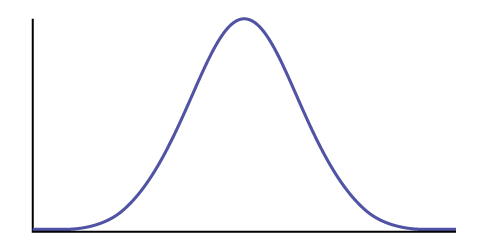2. P(0 < x < ____________) = ___________ (Use zero for the minimum value of x.)
1. Check student’s solution.
2. 3, 0.1979

Find the probability that a CD player will last between 2.8 and six years.

1. Sketch the situation. Label and scale the axes. Shade the region corresponding to the probability.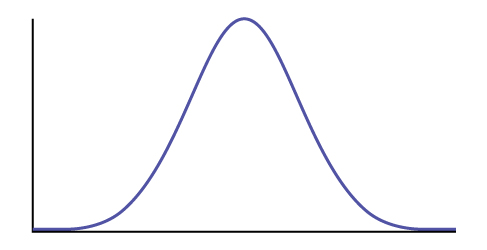2. P(__________ < x < __________) = __________

<!– <solution id=”id44443502″> Check student’s solution 2.8, 6, 0.7694 –>

An experiment with a probability of success given as 0.40 is repeated 100 times. Use the normal distribution to approximate the binomial distribution, and find the probability the experiment will have at least 45 successes.

0.154

An experiment with a probability of success given as 0.30 is repeated 90 times. Use the normal distribution to approximate the binomial distribution, and find the probability the experiment will have at least 22 successes.

0.874

An experiment with a probability of success given as 0.40 is repeated 100 times. Use the normal distribution to approximate the binomial distribution, and find the probability the experiment will have from 35 to 45 successes.

0.693

An experiment with a probability of success given as 0.30 is repeated 90 times. Use the normal distribution to approximate the binomial distribution, and find the probability the experiment will have from 26 to 30 successes.

0.346

An experiment with a probability of success given as 0.40 is repeated 100 times. Use the normal distribution to approximate the binomial distribution, and find the probability the experiment will have at most 34 successes.

0.110

An experiment with a probability of success given as 0.30 is repeated 90 times. Use the normal distribution to approximate the binomial distribution, and find the probability the experiment will have at most 34 successes.

0.946

A multiple choice test has a probability any question will be guesses correctly of 0.25. There are 100 questions, and a student guesses at all of them. Use the normal distribution to approximate the binomial distribution, and determine the probability at least 30, but no more than 32, questions will be guessed correctly.

0.071

A multiple choice test has a probability any question will be guesses correctly of 0.25. There are 100 questions, and a student guesses at all of them. Use the normal distribution to approximate the binomial distribution, and determine the probability at least 24, but no more than 28, questions will be guessed correctly.

0.347

### Homework

Use the following information to answer the next two exercises: The patient recovery time from a particular surgical procedure is normally distributed with a mean of 5.3 days and a standard deviation of 2.1 days.

What is the probability of spending more than two days in recovery?

1. 0.0580
2. 0.8447
3. 0.0553
4. 0.9420

<!– <solution id=”id12741946″> d –>

Use the following information to answer the next three exercises: The length of time it takes to find a parking space at 9 A.M. follows a normal distribution with a mean of five minutes and a standard deviation of two minutes.

Based upon the given information and numerically justified, would you be surprised if it took less than one minute to find a parking space?

1. Yes
2. No
3. Unable to determine

<!– <solution id=”id15290500″> a –>

Find the probability that it takes at least eight minutes to find a parking space.

1. 0.0001
2. 0.9270
3. 0.1862
4. 0.0668

d

Seventy percent of the time, it takes more than how many minutes to find a parking space?

1. 1.24
2. 2.41
3. 3.95
4. 6.05

<!– <solution id=”id16763246″> c –>

According to a study done by De Anza students, the height for Asian adult males is normally distributed with an average of 66 inches and a standard deviation of 2.5 inches. Suppose one Asian adult male is randomly chosen. Let X = height of the individual.

1. X ~ _____(_____,_____)
2. Find the probability that the person is between 65 and 69 inches. Include a sketch of the graph, and write a probability statement.
3. Would you expect to meet many Asian adult males over 72 inches? Explain why or why not, and justify your answer numerically.
4. The middle 40% of heights fall between what two values? Sketch the graph, and write the probability statement.
1. X ~ N(66, 2.5)
2. 0.5404
3. No, the probability that an Asian male is over 72 inches tall is 0.0082

IQ is normally distributed with a mean of 100 and a standard deviation of 15. Suppose one individual is randomly chosen. Let X = IQ of an individual.

1. X ~ _____(_____,_____)
2. Find the probability that the person has an IQ greater than 120. Include a sketch of the graph, and write a probability statement.
3. MENSA is an organization whose members have the top 2% of all IQs. Find the minimum IQ needed to qualify for the MENSA organization. Sketch the graph, and write the probability statement.

<!– <solution id=”eip-idp25493760″> N(100, 15) The probability that a person has an IQ greater than 120 is 0.0918. A person has to have an IQ over 130 to qualify for MENSA. The middle 50% of IQ scores falls between 89.95 and 110.05. –>

The percent of fat calories that a person in America consumes each day is normally distributed with a mean of about 36 and a standard deviation of 10. Suppose that one individual is randomly chosen. Let X = percent of fat calories.

1. X ~ _____(_____,_____)
2. Find the probability that the percent of fat calories a person consumes is more than 40. Graph the situation. Shade in the area to be determined.
3. Find the maximum number for the lower quarter of percent of fat calories. Sketch the graph and write the probability statement.
1. X ~ N(36, 10)
2. The probability that a person consumes more than 40% of their calories as fat is 0.3446.
3. Approximately 25% of people consume less than 29.26% of their calories as fat.

Suppose that the distance of fly balls hit to the outfield (in baseball) is normally distributed with a mean of 250 feet and a standard deviation of 50 feet.

1. If X = distance in feet for a fly ball, then X ~ _____(_____,_____)
2. If one fly ball is randomly chosen from this distribution, what is the probability that this ball traveled fewer than 220 feet? Sketch the graph. Scale the horizontal axis X. Shade the region corresponding to the probability. Find the probability.

<!– <solution id=”eip-idp105794048″> X ~ N(250, 50) The probability that a fly ball travels less than 220 feet is 0.2743. Eighty percent of the fly balls will travel less than 292 feet. –>

In China, four-year-olds average three hours a day unsupervised. Most of the unsupervised children live in rural areas, considered safe. Suppose that the standard deviation is 1.5 hours and the amount of time spent alone is normally distributed. We randomly select one Chinese four-year-old living in a rural area. We are interested in the amount of time the child spends alone per day.

1. In words, define the random variable X.
2. X ~ _____(_____,_____)
3. Find the probability that the child spends less than one hour per day unsupervised. Sketch the graph, and write the probability statement.
4. What percent of the children spend over ten hours per day unsupervised?
5. Seventy percent of the children spend at least how long per day unsupervised?
1. X = number of hours that a Chinese four-year-old in a rural area is unsupervised during the day.
2. X ~ N(3, 1.5)
3. The probability that the child spends less than one hour a day unsupervised is 0.0918.
4. The probability that a child spends over ten hours a day unsupervised is less than 0.0001.
5. 2.21 hours

In the 1992 presidential election, Alaska’s 40 election districts averaged 1,956.8 votes per district for President Clinton. The standard deviation was 572.3. (There are only 40 election districts in Alaska.) The distribution of the votes per district for President Clinton was bell-shaped. Let X = number of votes for President Clinton for an election district.

1. State the approximate distribution of X.
2. Is 1,956.8 a population mean or a sample mean? How do you know?
3. Find the probability that a randomly selected district had fewer than 1,600 votes for President Clinton. Sketch the graph and write the probability statement.
4. Find the probability that a randomly selected district had between 1,800 and 2,000 votes for President Clinton.
5. Find the third quartile for votes for President Clinton.

<!– <solution id=”eip-idp28319056″> X ~ N(1956.8, 572.3) This is a population mean, because all election districts are included. The probability that a district had less than 1,600 votes for President Clinton is 0.2676. 0.3798 Seventy-five percent of the districts had fewer than 2,340 votes for President Clinton. –>

Suppose that the duration of a particular type of criminal trial is known to be normally distributed with a mean of 21 days and a standard deviation of seven days.

1. In words, define the random variable X.
2. X ~ _____(_____,_____)
3. If one of the trials is randomly chosen, find the probability that it lasted at least 24 days. Sketch the graph and write the probability statement.
4. Sixty percent of all trials of this type are completed within how many days?
1. X = the distribution of the number of days a particular type of criminal trial will take
2. X ~ N(21, 7)
3. The probability that a randomly selected trial will last more than 24 days is 0.3336.
4. 22.77

Terri Vogel, an amateur motorcycle racer, averages 129.71 seconds per 2.5 mile lap (in a seven-lap race) with a standard deviation of 2.28 seconds. The distribution of her race times is normally distributed. We are interested in one of her randomly selected laps.

1. In words, define the random variable X.
2. X ~ _____(_____,_____)
3. Find the percent of her laps that are completed in less than 130 seconds.
4. The fastest 3% of her laps are under _____.
5. The middle 80% of her laps are from _______ seconds to _______ seconds.

<!– <solution id=”eip-idp63928720″> X = the distribution of race times that Terry Vogel produces X ~ N(129.71, 2.28) Terri completes 55.17% of her laps in less than 130 seconds. Terri completes 55.17% of her laps in less than 130 seconds. 124.4 and 135.02 –>

Thuy Dau, Ngoc Bui, Sam Su, and Lan Voung conducted a survey as to how long customers at Lucky claimed to wait in the checkout line until their turn. Let X = time in line. (Figure) displays the ordered real data (in minutes):

 0.5 4.25 5 6 7.25 1.75 4.25 5.25 6 7.25 2 4.25 5.25 6.25 7.25 2.25 4.25 5.5 6.25 7.75 2.25 4.5 5.5 6.5 8 2.5 4.75 5.5 6.5 8.25 2.75 4.75 5.75 6.5 9.5 3.25 4.75 5.75 6.75 9.5 3.75 5 6 6.75 9.75 3.75 5 6 6.75 10.75
1. Calculate the sample mean and the sample standard deviation.
2. Construct a histogram.
3. Draw a smooth curve through the midpoints of the tops of the bars.
4. In words, describe the shape of your histogram and smooth curve.
5. Let the sample mean approximate μ and the sample standard deviation approximate σ. The distribution of X can then be approximated by X ~ _____(_____,_____)
6. Use the distribution in part e to calculate the probability that a person will wait fewer than 6.1 minutes.
7. Determine the cumulative relative frequency for waiting less than 6.1 minutes.
8. Why aren’t the answers to part f and part g exactly the same?
9. Why are the answers to part f and part g as close as they are?
10. If only ten customers has been surveyed rather than 50, do you think the answers to part f and part g would have been closer together or farther apart? Explain your conclusion.
1. mean = 5.51, s = 2.15
2. Check student’s solution.
3. Check student’s solution.
4. Check student’s solution.
5. X ~ N(5.51, 2.15)
6. 0.6029
7. The cumulative frequency for less than 6.1 minutes is 0.64.
8. The answers to part f and part g are not exactly the same, because the normal distribution is only an approximation to the real one.
9. The answers to part f and part g are close, because a normal distribution is an excellent approximation when the sample size is greater than 30.
10. The approximation would have been less accurate, because the smaller sample size means that the data does not fit normal curve as well.

Suppose that Ricardo and Anita attend different colleges. Ricardo’s GPA is the same as the average GPA at his school. Anita’s GPA is 0.70 standard deviations above her school average. In complete sentences, explain why each of the following statements may be false.

1. Ricardo’s actual GPA is lower than Anita’s actual GPA.
2. Ricardo is not passing because his z-score is zero.
3. Anita is in the 70th percentile of students at her college.

<!– <solution id=”eip-idp7493680″> If the average GPA is less at Anita’s school than it is at Ricardo’s, then Ricardo’s actual score could be higher. Passing can be defined differently at different schools. Also, since Ricardo’s z-score is 0, his GPA is actually the average for his school, which is typically a passing GPA. Anita’s percentile is higher than the 70th percentile. –>

An expert witness for a paternity lawsuit testifies that the length of a pregnancy is normally distributed with a mean of 280 days and a standard deviation of 13 days. An alleged father was out of the country from 240 to 306 days before the birth of the child, so the pregnancy would have been less than 240 days or more than 306 days long if he was the father. The birth was uncomplicated, and the child needed no medical intervention. What is the probability that he was NOT the father? What is the probability that he could be the father? Calculate the z-scores first, and then use those to calculate the probability.

<!– <solution id=”eip-102″> For x = 240, X−μ σ = 240−280 13 =−3.0769  For x = 306, 306−280 13 =2 P(240 < x < 306) = P(–3.0769 < z < 2) = normalcdf(–3.0769,2,0,1) = 0.9762. According to the scenario given, this means that there is a 97.62% chance that he is not the father. To answer the second part of the question, there is a 1 – 0.9762 = 0.0238 = 2.38% chance that he is the father. –>

A NUMMI assembly line, which has been operating since 1984, has built an average of 6,000 cars and trucks a week. Generally, 10% of the cars were defective coming off the assembly line. Suppose we draw a random sample of n = 100 cars. Let X represent the number of defective cars in the sample. What can we say about X in regard to the 68-95-99.7 empirical rule (one standard deviation, two standard deviations and three standard deviations from the mean are being referred to)? Assume a normal distribution for the defective cars in the sample.

• n = 100; p = 0.1; q = 0.9
• μ = np = (100)(0.10) = 10
• σ ==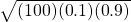= 3
1.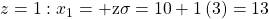and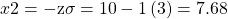of the defective cars will fall between seven and 13.
2.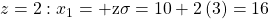and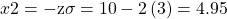of the defective cars will fall between four and 16
3.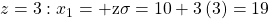and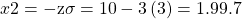of the defective cars will fall between one and 19.

We flip a coin 100 times (n = 100) and note that it only comes up heads 20% (p = 0.20) of the time. The mean and standard deviation for the number of times the coin lands on heads is µ = 20 and σ = 4 (verify the mean and standard deviation). Solve the following:

1. There is about a 68% chance that the number of heads will be somewhere between ___ and ___.
2. There is about a ____chance that the number of heads will be somewhere between 12 and 28.
3. There is about a ____ chance that the number of heads will be somewhere between eight and 32.

<!– <solution id=”eip-171″> There is about a 68% chance that the number of heads will be somewhere between 16 and 24. z = ±1: x1 = µ + zσ = 20 + 1(4) = 24 and x2 = µ-zσ = 20 – 1(4) = 16. There is about a 95% chance that the number of heads will be somewhere between 12 and 28. For this problem: normalcdf(12,28,20,4) = 0.9545 = 95.45% There is about a 99.73% chance that the number of heads will be somewhere between eight and 32. For this problem: normalcdf(8,32,20,4) = 0.9973 = 99.73%. –>

A ?1 scratch off lotto ticket will be a winner one out of five times. Out of a shipment of n = 190 lotto tickets, find the probability for the lotto tickets that there are

1. somewhere between 34 and 54 prizes.
2. somewhere between 54 and 64 prizes.
3. more than 64 prizes.
• n = 190; p == 0.2; q = 0.8
• μ = np = (190)(0.2) = 38
• σ ==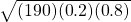= 5.5136
1. For this problem: P(34 < x < 54) = 0.7641
2. For this problem: P(54 < x < 64) = 0.0018
3. For this problem: P(x > 64) = 0.0000012 (approximately 0)

Facebook provides a variety of statistics on its Web site that detail the growth and popularity of the site.

On average, 28 percent of 18 to 34 year olds check their Facebook profiles before getting out of bed in the morning. Suppose this percentage follows a normal distribution with a standard deviation of five percent.

<!– <solution id=”eip-11″> X = the percent of 18 to 34-year-olds who check Facebook before getting out of bed in the morning. X ~ N(28, 5) P(x ≥ 30) = 0.3446; normalcdf(30,1EE99,28,5) = 0.3446 invNorm(0.95,0.28,0.05) = 0.3622.95% of the percent of 18 to 34 year olds who check Facebook before getting out of bed in the morning is at most 36.22%. P(25 < x < 55). P(25 < x < 55) = normalcdf(25,55,28,5) = 0.7257(0.7257)(400) = 290.28 Find the probability that the percent of 18 to 34-year-olds who check Facebook before getting out of bed in the morning is at least 30. –>

A hospital has 49 births in a year. It is considered equally likely that a birth be a boy as it is the birth be a girl.

1. What is the mean?
2. What is the standard deviation?
3. Can this binomial distribution be approximated with a normal distribution?
4. If so, use the normal distribution to find the probability that at least 23 of the 49 births were boys.
1. 24.5
2. 3.5
3. Yes
4. 0.67

Historically, a final exam in a course is passed with a probability of 0.9. The exam is given to a group of 70 students.

1. What is the mean of the binomial distribution?
2. What is the standard deviation?
3. Can this binomial distribution be approximate with a normal distribution?
4. If so, use the normal distribution to find the probability that at least 60 of the students pass the exam?
1. 63
2. 2.5
3. Yes
4. 0.88

A tree in an orchard has 200 oranges. Of the oranges, 40 are not ripe. Use the normal distribution to approximate the binomial distribution, and determine the probability a box containing 35 oranges has at most two oranges that are not ripe.

0.02

In a large city one in ten fire hydrants are in need of repair. If a crew examines 100 fire hydrants in a week, what is the probability they will find nine of fewer fire hydrants that need repair? Use the normal distribution to approximate the binomial distribution.

0.37

On an assembly line it is determined 85% of the assembled products have no defects. If one day 50 items are assembled, what is the probability at least 4 and no more than 8 are defective. Use the normal distribution to approximate the binomial distribution.

0.50